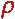Math & Science Home | Proficiency Tests | Mathematical Thinking in Physics | Aeronauts 2000

 CONTENTS Introduction Fermi's Piano Tuner Problem How Old is Old? If the Terrestrial Poles were to Melt... Sunlight Exerts Pressure Falling Eastward What if an Asteroid Hit the Earth Using a Jeep to Estimate the Energy in Gasoline How do Police Radars really work? How "Fast" is the Speed of Light? How Long is a Light Year? How Big is a Trillion? "Seeing" the Earth, Moon, and Sun to Scale Of Stars and Drops of Water If I Were to Build a Model of the Cosmos... A Number Trick Designing a High Altitude Balloon Pressure in the Vicinity of a Lunar Astronaut Space Suit due to Outgassing of Coolant Water Calendar Calculations Telling Time by the Stars - Sidereal Time Fields, an Heuristic Approach The Irrationality ofThe Irrationality ofThe Number (i)i Estimating the Temperature of a Flat Plate in Low Earth Orbit Proving that (p)1/n is Irrational when p is a Prime and n>1 The Transcendentality ofIdeal Gases under Constant Volume, Constant Pressure, Constant Temperature and Adiabatic Conditions Maxwell's Equations: The Vector and Scalar Potentials A Possible Scalar Term Describing Energy Density in the Gravitational Field A Proposed Relativistic, Thermodynamic Four-Vector Motivational Argument for the Expression-eix=cosx+isinx Another Motivational Argument for the Expression-eix=cosx+isinx Calculating the Energy from Sunlight over a 12 hour period Calculating the Energy from Sunlight over actual full day Perfect Numbers-A Case Study Gravitation Inside a Uniform Hollow Sphere Further note on Gravitation Inside a Uniform Hollow Sphere Pythagorean Triples Black Holes and Point Set Topology Additional Notes on Black Holes and Point Set Topology Field Equations and Equations of Motion (General Relativity) The observer in modern physics A Note on the Centrifugal and Coriolis Accelerations as Pseudo Accelerations - PDF File On Expansion of the Universe - PDF File

## Designing a High Altitude Balloon

Problem:
Design a gas balloon to carry an instrument package in the earth's atmosphere at an altitude of 90 km. Specify how large the balloon will have to be and the mass of gas it will require to maintain this altitude if:

1. It uses helium (He).
2. It uses hydrogen (H2).

Assume that the ideal gas law holds at the desired altitude, that the inflated balloon is spherical, and that the total payload mass (balloon + instrument package) is 10 kg.

Solution:
Let's assume that:

1. The buoyant force on the balloon is equal to the weight of the (fluid) atmosphere displaced.
2. The ideal gas law holds in the form p = nkT
1. where n is the number density in m-3, k is Boltzmann's constant (k = 1.38 X 10-23 j/K), T is the absolute temperature, and p is the pressure.

2. Atmospheric conditions at an altitude of 90 km are:
 Temperature: T = 187 K Pressure: p = 1.38 x 10-3 Torr Density:A = 3.42 x 10-6 kg/m3

(ref.: U. S. Standard Atmosphere, 1976).

Then:

1. In order for the balloon to stay aloft, we know that the buoyant force must equal the weight of the balloon and instrument package plus the weight of the (hydrogen or helium) gas inside the balloon. IfAVBg is the weight of displaced atmosphere, then
1.AVBg = (10 kg)g +GVBg

 orAVB = 10 kg +GVB 1

whereG is the gas density inside the balloon, VB is volume of the balloon, and g is the local gravitational acceleration.

2. We use the ideal gas law to obtain the gas density inside the balloon. For helium: p = nkT = (G/m)kT

with m = 6.68 x 10-27 kg. Set p = 1.38 X 10-3 torr = 1.82 x 10-6 atm = 0.18 nt/m2, and T = 187 K, then solve forG:G = 4.66 x 10-7 kg/m3 2

This number represents the density of helium at the pressure and temperature conditions stated for 90 km altitude. It is assumed to be the helium gas density inside the balloon as well.8

3. We now use eq. 2 in eq. 1 to calculate the volume of the balloon:
 VB = 3.39 x 106 m3 3

and we use eq. 3 with eq. 2 to calculate the mass of helium required:

 mHe = 1.58 kg 4

If the balloon is assumed to be spherical, then

VB = (4/3)rB3.

where rB is the balloon radius. Using the value of VB in eq. 3, we find that the radius of the balloon is

 rB = 93 m 5

If hydrogen is used instead of helium, then we set mH2 = 1.67 x 10-27 kg in the ideal gas law, and repeat the above procedure to findG = 1.16 x 10-7 kg/m3 2a.
 VB = 3.03 x 106 m3 3a.
 mH2 = 0.35 kg 4a.
 rB = 90 m 5a.

8 Thus, the interior and exterior pressures are equal. This assumption is equivalent to saying that the balloon is inflated without stretching; i.e., that there is no tension in its material.